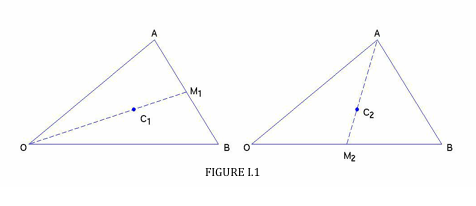$$\require{cancel}$$

# 1.2: Plane Triangular Lamina

•• Contributed by Jeremy Tatum
• Emeritus Professor (Physics & Astronomy) at University of Victoria

Definition: A median of a triangle is a line from a vertex to the midpoint of the opposite side.

Theorem I. The three medians of a triangle are concurrent (meet at a single, unique point) at a point that is two-thirds of the distance from a vertex to the midpoint of the opposite side.

Theorem II. The centre of mass of a uniform triangular lamina (or the centroid of a triangle) is at the meet of the medians.

The proof of I can be done with a nice vector argument (Figure I.1):

Let $$\bf{\text{A}}$$ , $$\bf{\text{B}}$$ be the vectors $$\text{OA}$$ , $$\text{OB}$$ . Then $$\bf{\text{A+B}}$$ is the diagonal of the parallelogram of which $$OA$$ and $$\text{OB}$$ are two sides, and the position vector of the point $$\text{C}_{1}$$ is $$\frac{1}{3}(\bf{\text{A+B}})$$ .

To get $$\text{C}_{2}$$, we see that

$${\bf C}_2 = {\bf A} + \dfrac{2}{3}(\text{AM}_2) = {\bf A} + \dfrac{2}{3}({\bf M_2 - A}) = {\bf A} + \dfrac{2}{3}(\dfrac{1}{2}{\bf B- A}) = \dfrac{1}{3}( {\bf A+B})$$Thus the points $$\text{C}_{1}$$ and $$\text{C}_{2}$$ are identical, and the same would be true for the third median, so Theorem I is proved.

Now consider an elemental slice as in Figure I.2. The centre of mass of the slice is at its mid-point. The same is true of any similar slices parallel to it. Therefore the centre of mass is on the locus of the mid-points - i.e. on a median. Similarly, it is on each of the other medians, and Theorem II is proved.That needed only some vector geometry. We now move on to some calculus.